# UniRel: Unified Representation and Interaction for Joint Relational Triple Extraction

## Basic Idea

1. 实体表示和关系表示是异质的.
2. 异质的建模了实体 - 实体间交互和实体 - 关系间交互.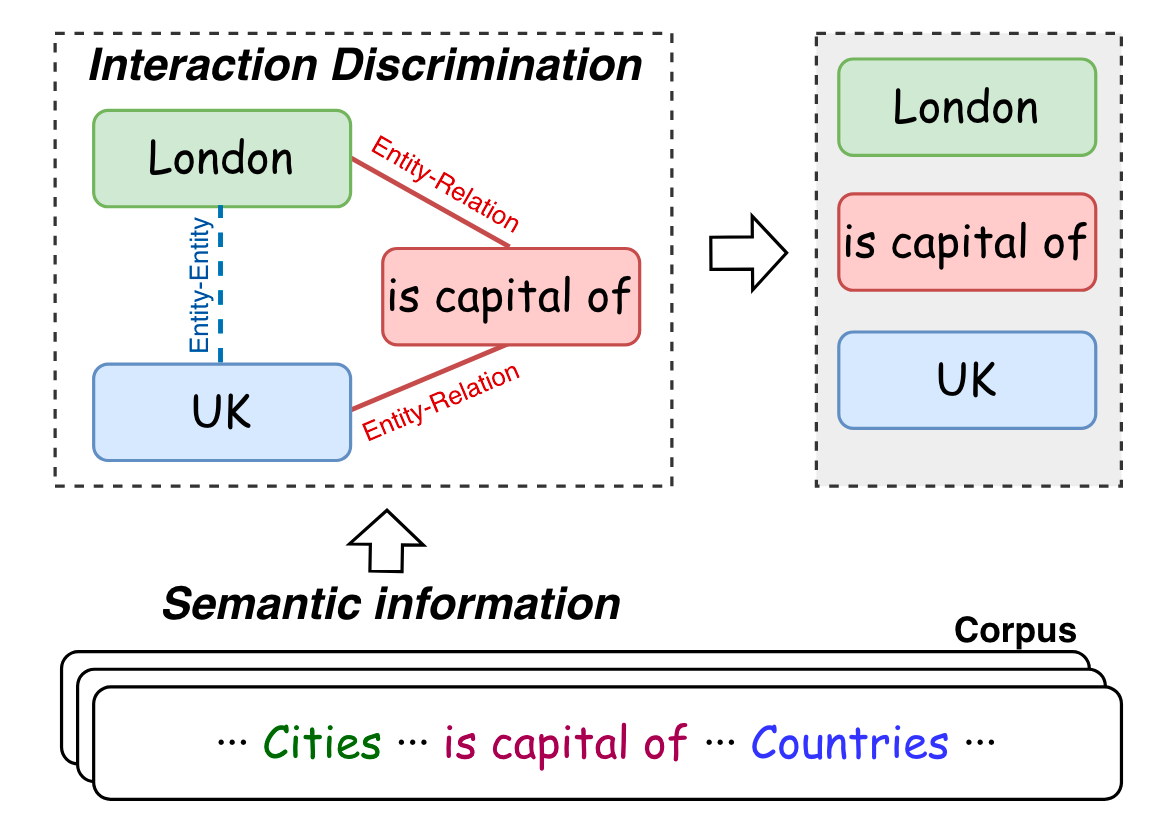## UniRel

### Unified Representation

$$\begin{gathered} T=\operatorname{Concat}\left(T_s, T_p\right) \\ H=E[T] \end{gathered}$$

$$\operatorname{Attention}(Q, K, V)=\operatorname{softmax}\left(\frac{Q K^T}{\sqrt{d_h}}\right) V$$

### Unified Interaction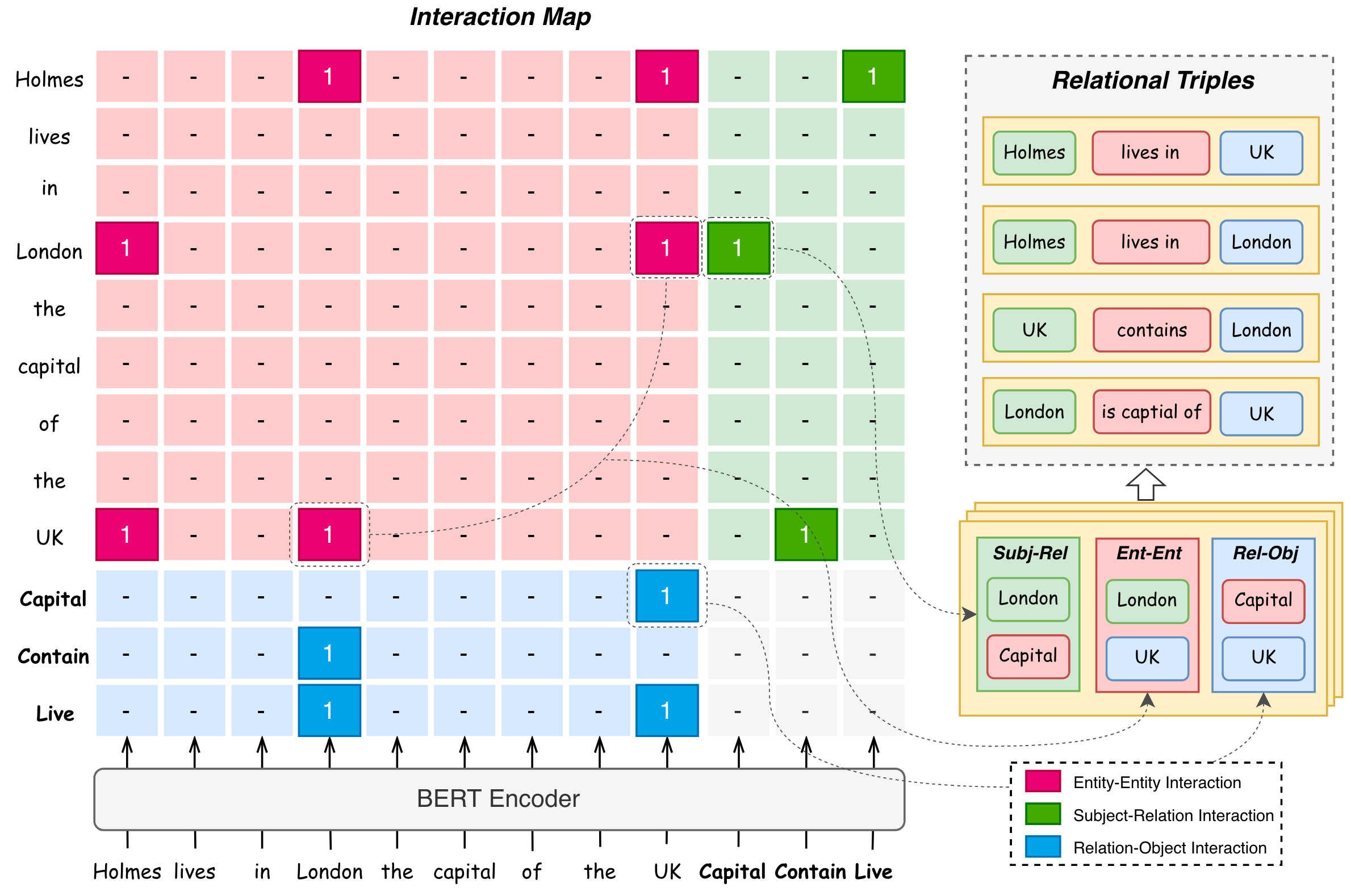• 红色区域: Entity - Entity Interaction.
• 绿色区域: Subject - Relation Interaction.
• 蓝色区域: Relation - Object Interaction.

#### Entity - Entity Interaction

$$I_e\left(e_a, e_b\right)= \begin{cases}\text { True } & \left(e_a, r, e_b\right) \in T \text { or } \\\ & \left(e_b, r, e_a\right) \in T, \exists r \in R \\\ \text { False } & \text { otherwise }\end{cases}$$

Entity - Entity Interaction 建模了实体之间的对齐关系(实体之间的成对关系).

#### Entity - Relation Interaction

\begin{aligned} & I_r(e, r)= \begin{cases}\text { True } & (e, r, o) \in T, \exists o \in E \\ \text { False } & \text { otherwise }\end{cases} \\ & I_r(r, e)= \begin{cases}\text { True } & (s, r, e) \in T, \exists s \in E \\ \text { False } & \text { otherwise }\end{cases} \end{aligned}

#### Interaction Discrimination

$$\mathbf{I}=\operatorname{sigmoid}\left(\frac{1}{T} \sum_t^T \frac{Q_t K_t^T}{\sqrt{d_h}}\right)$$

#### Training and Decoding

##### Loss Function

\begin{aligned} \mathcal{L}=-\frac{1}{(N+M)^2} \sum_i^{N+M} \sum_j^{N+M}\left(\mathbf{I}_{i, j}^\ast \log \mathbf{I}_{i, j}\right. \left.+\left(1-\mathbf{I}_{i, j}^\ast\right) \log \left(1-\mathbf{I}_{i, j}\right)\right) \end{aligned}

$\mathbf{I}^\ast$ 为交互图矩阵中的Ground Truth.

##### Decoding1. 根据右侧的绿色框框(Subj - Rel)和下面的蓝色框框(Rel - Obj)分别解码出每种关系$r$ 对应的Subject $e_i$ 和Object $e_j$. 即抽取出$(e_{i,}r), (r, e_j)$.
2. 根据上一步找到的所有实体$e_i, e_j$, 穷举出所有的实体对$(e_{p}, e_q)$, 结合左上角的红色框框(Ent - Ent), 查看是否有对应的$(e_{p,}e_q)$ 为1.
3. 如果在红色框框里有$(e_{p,}e_q)$ 为1, 则构成三元组$(e_{i},r, e_j)$. 即利用Subj - Rel $(e_{i},r)$, Ent - Ent $(e_{i,}e_j)$, Rel - Obj $(r, e_j)$ 共同形成三元组$(e_{i,}r, e_j)$.

1. 首先从右侧的绿色框框拿到Subj - Rel, 有(Holmes, Live), (London, Capital), (UK, Contain).
2. 从下面的蓝色框框拿到Rel - Obj, 有(Capital, London), (Live, London), (Capital, UK), (Live, UK).
3. 然后穷举出所有的实体对, 并结合红色框框Ent - Ent进行检查, 有效的有(Holmes, London), (Holmes, UK), (UK, London), (London, UK) 这四个实体对.
4. 结合Subj - Rel, Rel - Obj, Ent - Ent, 可以解码出关系三元组. 比如, Subj - Rel有(Holmes, Live), Rel - Obj有(Live, London), 同时Ent - Ent有(Holmes, London), 那么就可以解码出三元组(Holmes, Live, London). 与之类似的, 可以共同解码出(Holmes, Live, UK), (UK, Contain, London), (London, Capital, UK) 这其他三个三元组.

### Extended to Multi - token Entity Setting

1. 将实体识别从单Token识别扩展为边界识别. 即先将一张交互图变为两张, 来分别完成(Subject Head, Relation, Object Head)以及(Subject Tail, Relation, Object Head)的抽取.
2. 添加第三张关于每个实体的头尾交互图, 完成(Head, Relation, Tail)的抽取.
3. 对于每个关系, 将实体的头尾Token链接起来, 作为一个实体, 然后像之前一样解码.

## Experiments

### Datasets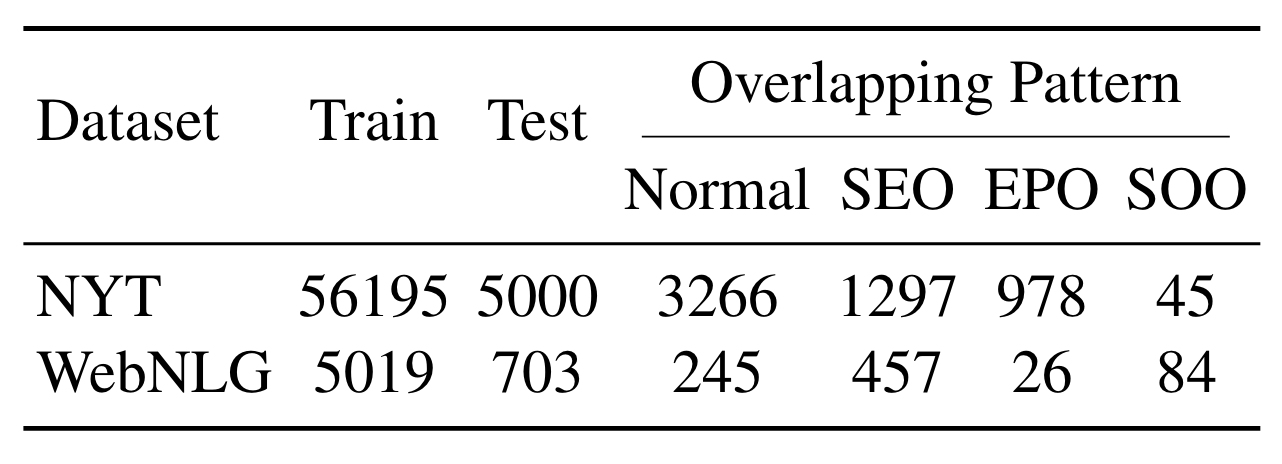### Results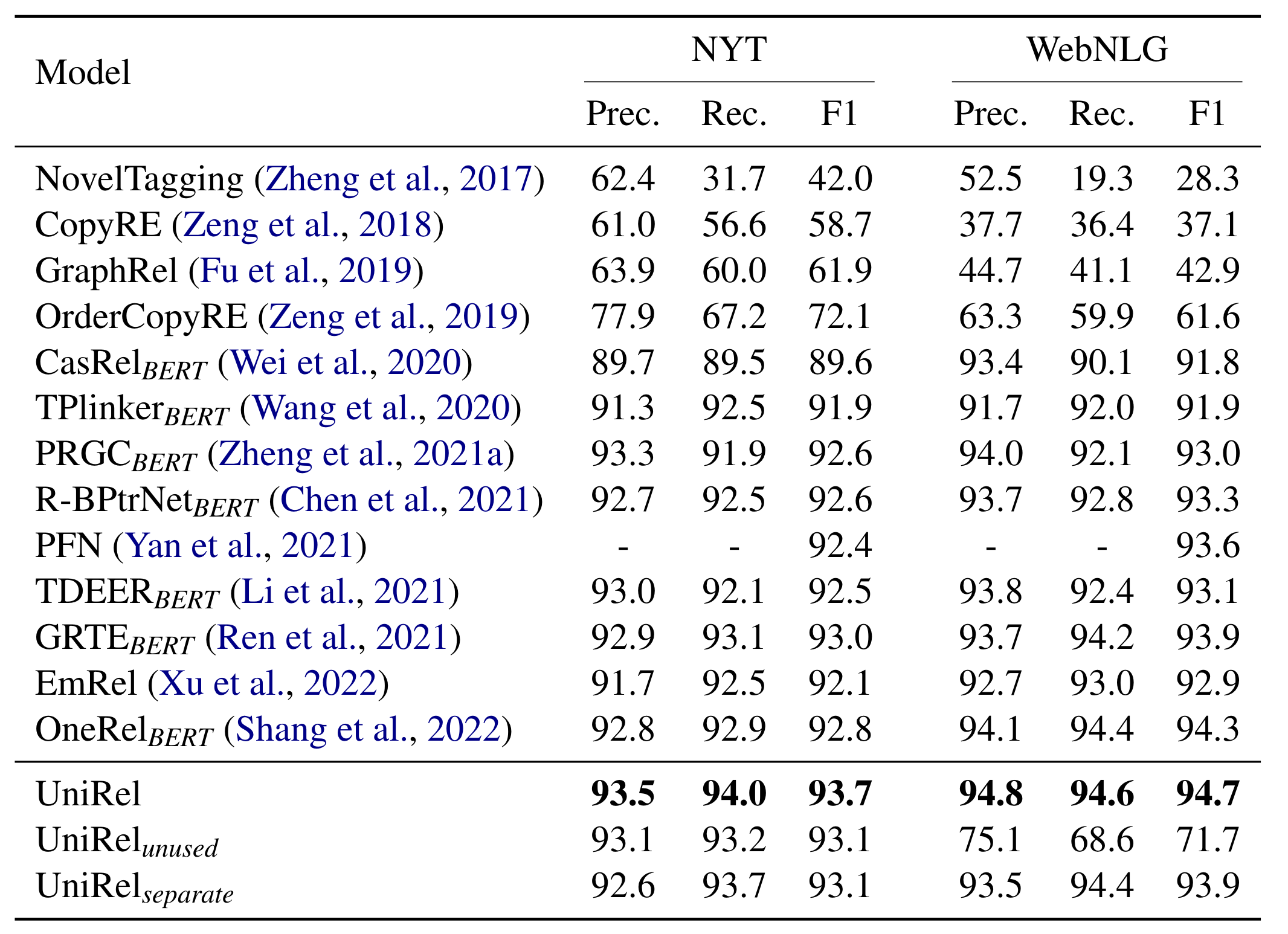• unused: 用BERT[unused*] Token来代替每种关系的语义Embedding, 也就是重新训练一个随机初始化的关系Embedding.
• separate: 用一个共享的BERT分别将输入的句子关系语义Token编码, 然后用不同的两个Transformer Layer获得对应的Q和K, 再将Q, K分别拼接后做点积预测.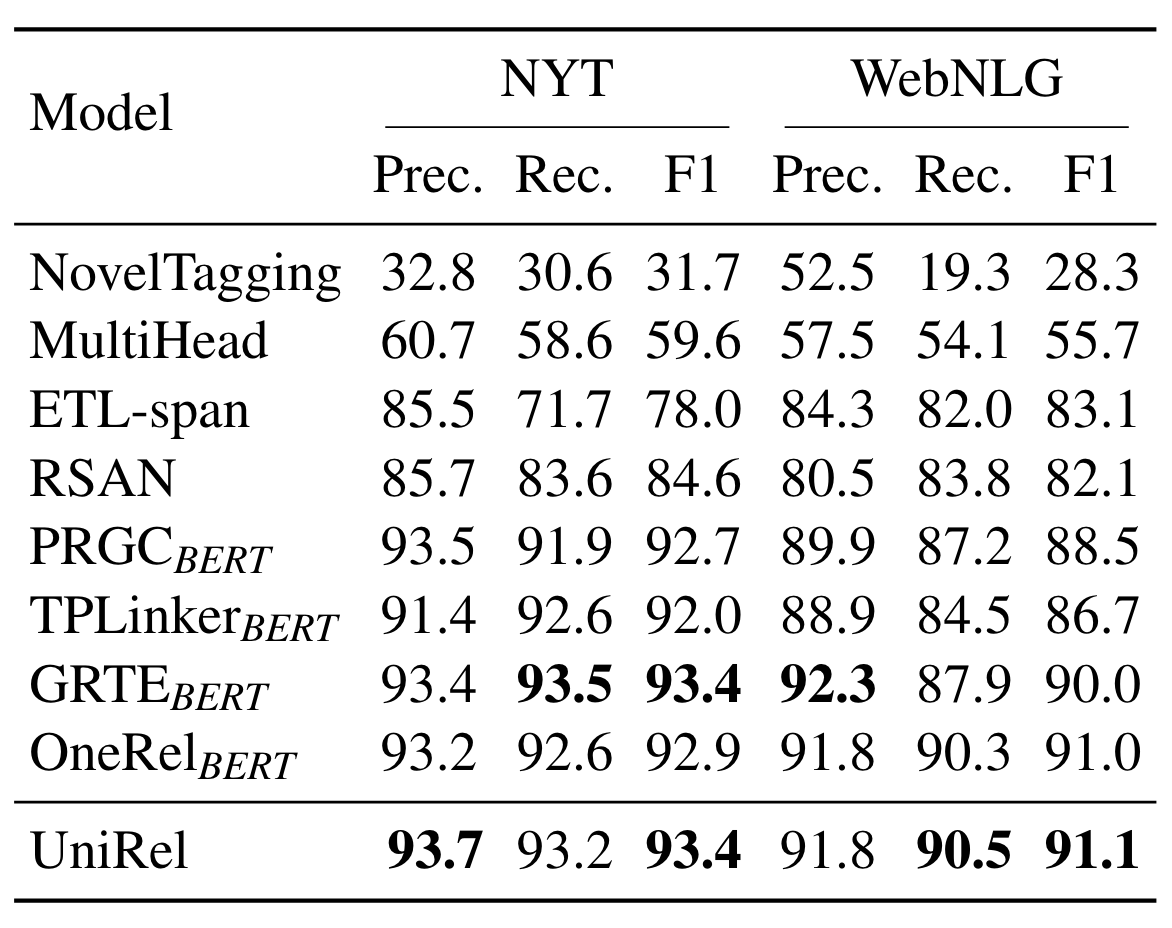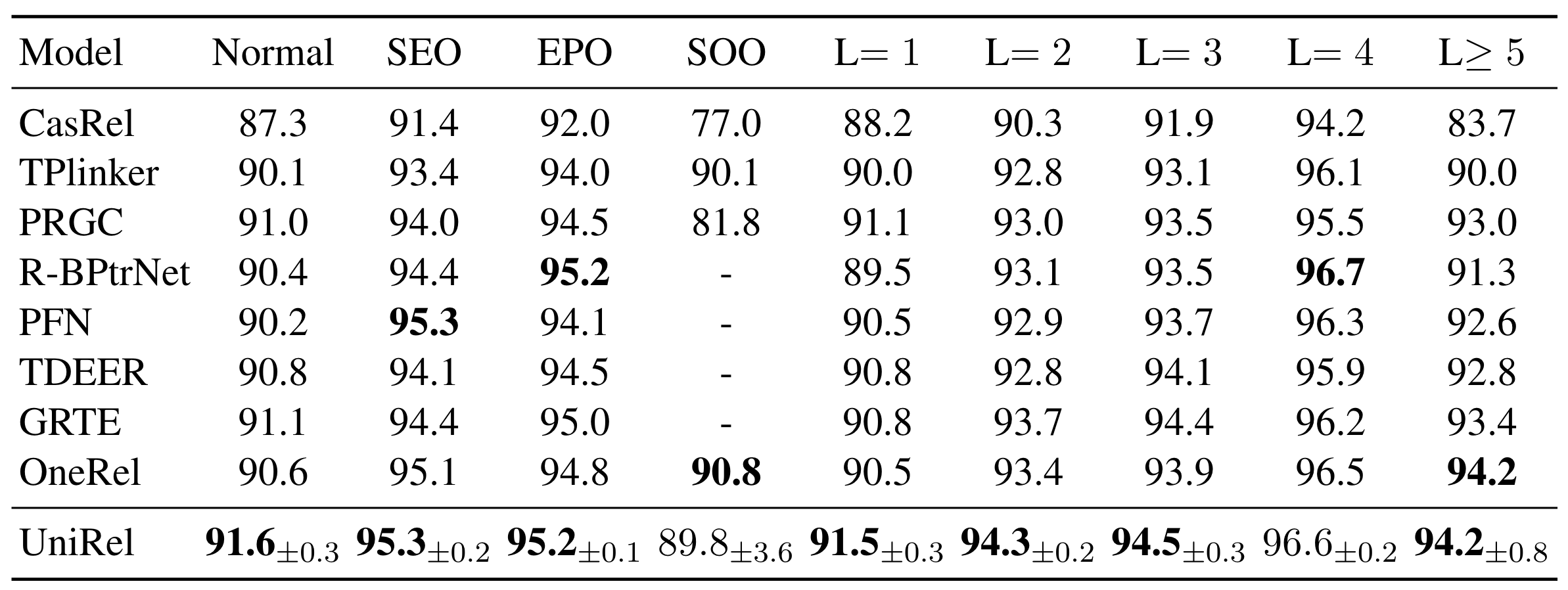### Analysis

#### Effect of the Unified Representation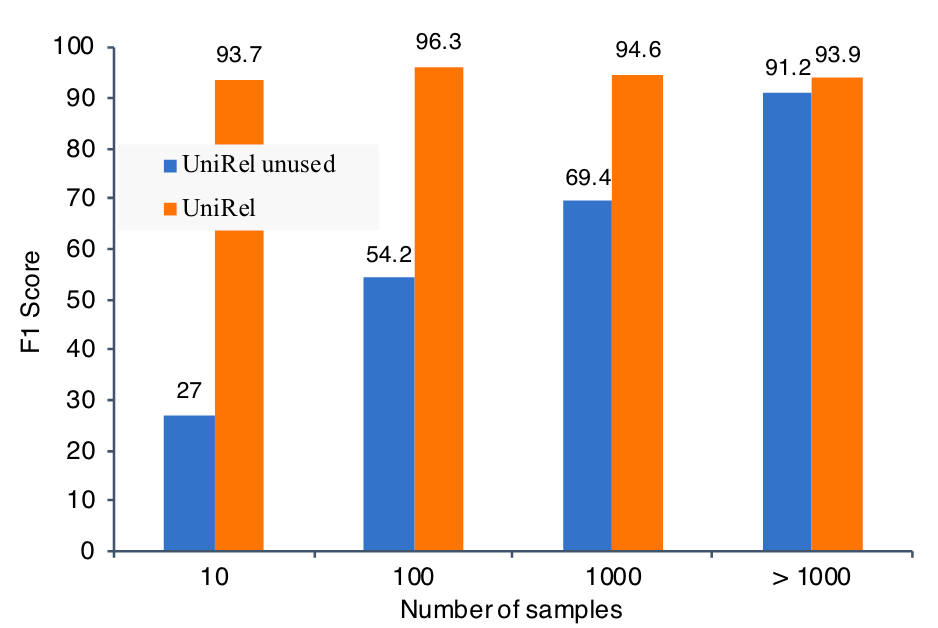F1明显的在关系所对应的样本数量增大后提升, 但UniRel在融入了一定的语义信息后, 即使是在>1000条样本的设置下, 比unused 自由学习结果还是要好一些.

#### Effect of the Unified Interaction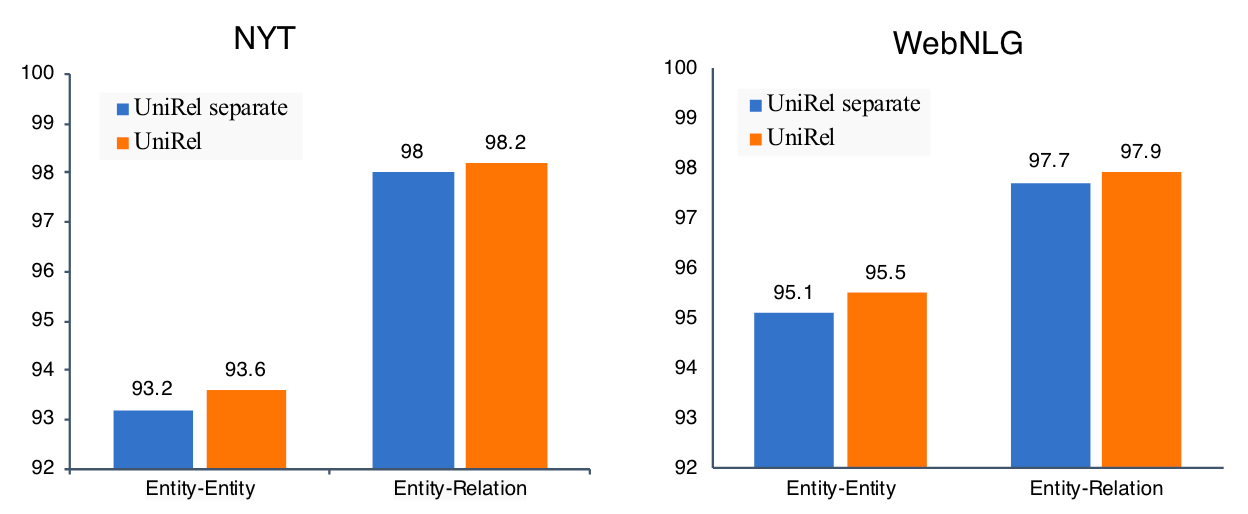#### Computational Efficiency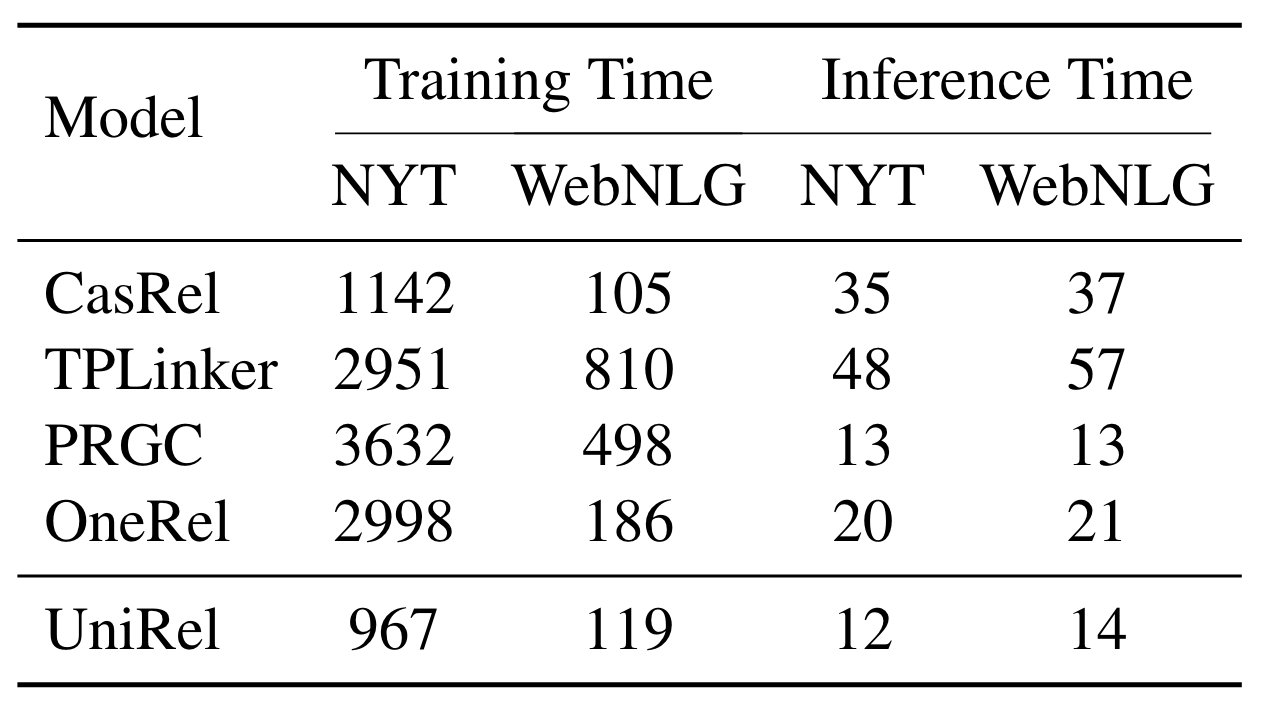#### Visualization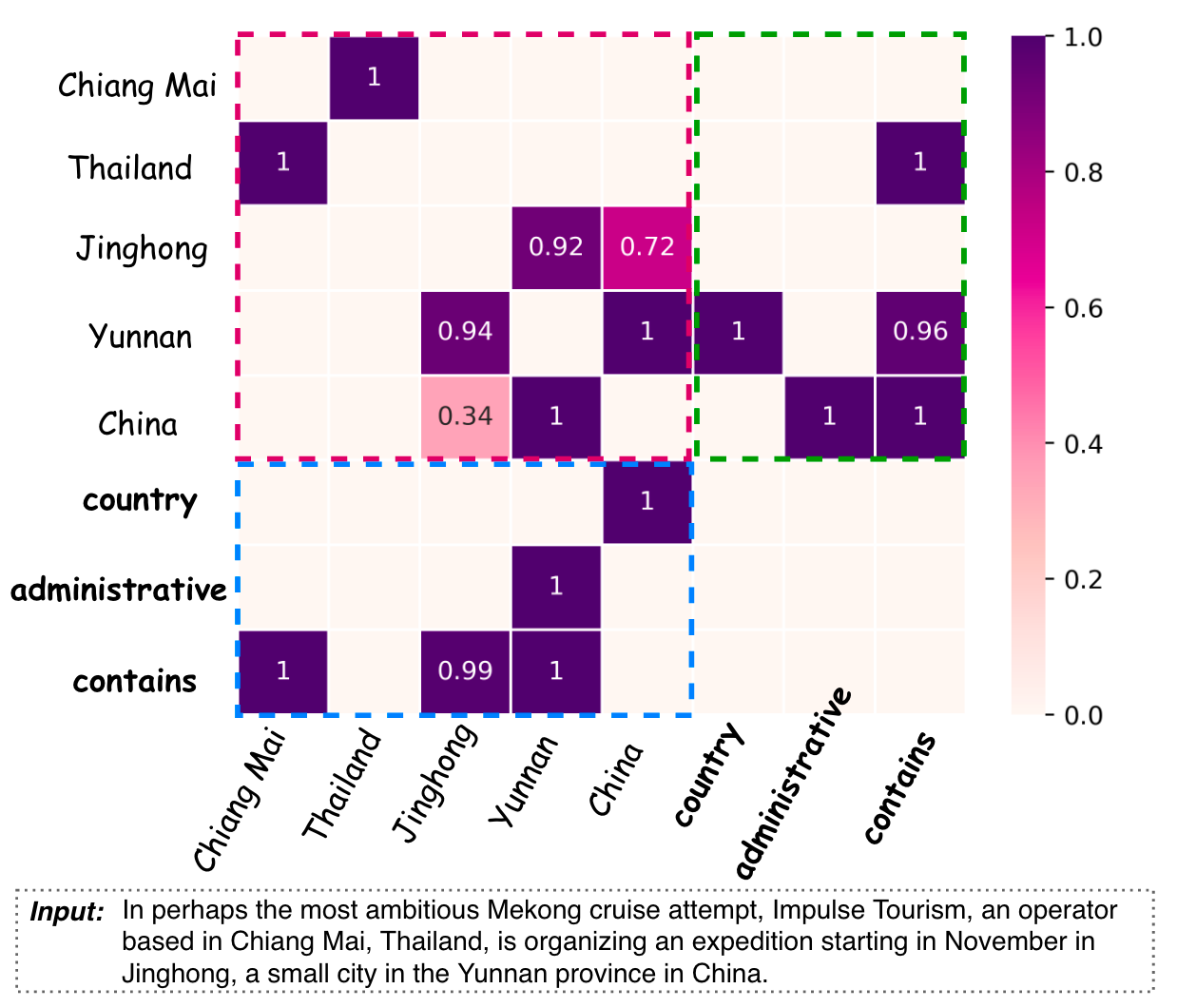## Summary

UniRel是一种表填充式的RTE方法, 通过将关系转化为语义Token, 把实体和关系变成了统一表示方法, 并且做完全同质建模, 效果达到了目前任务上真正的SOTA.

上一篇QIDN: Query-based Instance Discrimination Network for Relational Triple Extraction
Query-based Instance Discrimination Network for Relational Triple Extraction本文是论文Query-based Instance Discrimination Net
2023-02-10OneEE: A One-Stage Framework for Fast Overlapping and Nested Event Extraction
OneEE: A One-Stage Framework for Fast Overlapping and Nested Event Extraction本文是论文OneEE: A One-Stage Framework for Fast
2022-11-21
目录